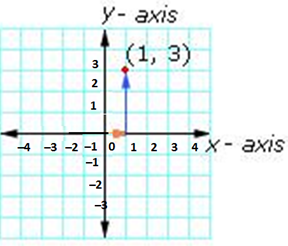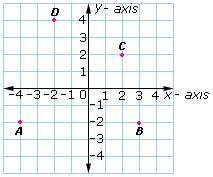Related Links

## Definition Of X-Coordinate

The first number in an ordered pair is the x-coordinate

### More About X-Coordinate

Another name for x-coordinate of a point is "abscissa"
The x-coordinate of a point tells how far to move left or right from the origin along the x-axis

### Examples of X-Coordinate

In the ordered pair (2, 4), the x-coordinate or abscissa is 2
In the ordered pair (- 1, 7), the x-coordinate or abscissa is - 1
To reach the point (1, 3), move 1 unit to the left from the origin and then 3 units up### Solved Example on X-Coordinate

#### Ques: Which of the points has - 4 as the x-coordinate?##### Choices:

A. D
B. C
C. B
D. A
Correct answer: D

#### Solution:

Step 1: The ordered pair for the point D is (- 2, 4). So, D has -2 as the x-coordinate
Step 2: The ordered pair for the point C is (2, 2). So, C has 2 as the x-coordinate
Step 3: The ordered pair for the point B is (3, - 2). So, B has 3 as the x-coordinate
Step 4: The ordered pair for the point A is (- 4, - 2). So, A has -4 as the x-coordinate
Step 5: So, the point A has -4 as the x-coordinate.